# Black-box Identification and Validation of an Induction Motor in an Experimental Application

Black-box Identification and Validation of an Induction Motor in an Experimental Application
School of Railway Engineering, Iran University of Science and Technology, Tehran 16846-13114, Iran

Department of Electrical Engineering, Iran University of Science and Technology, Tehran 16846-13114, Iran

Corresponding Author Email:
rashidi.atena.67@gmail.com
Page:
255-263
|
DOI:
https://doi.org/10.18280/ejee.210219
25 January 2019
|
Accepted:
20 March 2019
|
Published:
30 April 2019
| Citation

OPEN ACCESS

Abstract:

This article presents a simple approach to identify an induction motor model which is used in a traction control system for the purpose of slip prevention. In this study, an identified model has been extracted by real and identified parameters of the induction motor in the MATLAB, Simulink; as the purpose of this study is to identify a linear model for the induction motor, the speed control loops have been opened, therefore d-q voltages have been designed as the exiting inputs. After several considerations and examination, ARMAX model has been selected as a mathematical model in the identification process, then the open loop identified model has been replaced in the closed-loop structure, and by using the real data measurements, obtained from the closed-loop system in the Advanced Motion Control Laboratory, the open loop extracted model is verified.

Keywords:

slip controller, ARMAX, PRBS, dq voltages, drive, FOC, identification algorithm

1. Introduction

One of the most important milestones of recent decades is the advent of the traction control system for an electric vehicle which has played a prominent role in improving the transportation systems. Traction controllers are used to controlling a vehicle under adverse external conditions . In the railway vehicle, increasing the slip ratio decreases the friction between the rail and the wheel, and consequently, it reduces the tractive effort, which is not desired [2-3]. In the railway, slip occurs when the friction between the rail and wheel is low and, the peripheral speed of the wheel is more than the longitudinal velocity of the rail vehicle . According to this statement, the slip ratio in traction mode is defined as follows:

$\lambda=\frac{r \omega-v}{v}$

where $\lambda$ is the slip ratio, $\mathcal{V}$ is the longitudinal velocity of the train, $\omega$ is the angular velocity of the wheel and is the rolling radius of the wheel. In order to prevent wheel slippage and intensify traction forces, locomotive traction control systems are widely used to maximize the friction coefficient between the rail and wheels. Using a traction control system, the slip ratio keeps in an optimal amount, improving the tractive effort which causes a reduction in both energy consumption and maintenance costs. By maximizing the tractive force between the wheels and rail, a traction controller prevents the wheel from slipping [2-3]. The induction motors have been widely applied as the traction motor because of the low maintenance, low cost . Advances in power electronics and microprocessor technology make it feasible to use the induction motors in place of DC motor and synchronous motors in a wide range of servo applications.

In order to get a traction control system had the desired performance and kept the slip ration in an optimal value, it is indispensable to bring up an accurate model of the induction motor as an electromechanical actuator in the traction control system. Modeling and simulation have been widely used both in the designer and user stage for predicting and studying steady-state and transient behavior of the induction motors . Induction motor identification is classified into two categories. The first one is related to the parameter identification and the second one is concerned with black, gray box modeling of an induction motor.

1.1 Parameters identification of an induction motor

Numerous methods for induction motor parameter identification have been developed.  These methods are categorized to on-line and off-line parameter estimation methods for application in high-performance drives . The offline methods perform the parameter identification while the motor is at a standstill and no torque is required from the mechanical load. This kind of procedure is usually performed during the initialization of the motion control process [7-11]. Differently, the online approaches execute the IM identification while the electric drive is operating normally, i.e. while motion control is performed [12-17]. The main advantage of this kind of solution with respect to the previous ones is the capability of tracking the slow variation of motor parameters during operating conditions .

1.2 Black or gray box modeling of an induction motor

In these works, the transfer functions representing the motor drive model are estimated using identification algorithms. This approach is considered the system as a black box [19-21]. Modeling and simulation are basic stages for the designer and user to predict and studies the transient and steady-state of a certain process [20-22]. Arrofiq et al.  Suggested an identification of a system arranged by a variable speed drive, an induction motor, and a dynamometer. This system has two inputs, variable speed drive control voltage and load applied and its output is speed. In this application, some model structures such as ARX, ARMAX, Output-Error (OE), Box Jenkins (BJ), state-space model are considered. In the end, the best fit model is selected. The identification results in three poles, single zero with dead time process-model.

Identified the black-box model of an induction motor to obtain a linear model . They assumed the noise/disturbance-free model structure (ARMAX) to identify the induction motor by a pseudo-random binary sequence (PRBS) chosen as the input signal. In this application voltage signal sent to the industrial frequency inverter is the input and motor speed is the output signal.  In some researches neural network was used to identify a model such as ([21, 23]). Akbari et al.  used the recurrent neural network introduced by Elman  to identify a dynamic model of an induction motor in all states and conditions whether transient or steady. They used the load torque as the input and the q current as the output to learn the neural network.

Mohamed and Koivo  proposed a neural network ARX model. They have used a PRBS signal for Voltage signal which should be sent to the industrial frequency inverter as the input signal and measured motor speed as the output signal. Paper  proposed a gray-box identification of an induction motor model. Researcher  used PEM identification algorithms to derive the transfer function. In this experimental application, the outputs of the current PI controllers in FOC structure are the input signal of the identification process and the speed and dq currents are the outputs of it.

This study deliberated over identifying the interdependent dynamic linear models for an induction motor as an actuator in a traction system, simulated in a setup. This setup is made by researchers in . In order to analyze the slip control in the traction mode in a railway vehicle, Sadr et al.  introduced a hardware setup, simulating the movement of the electrical train wheel on the rail. They used a mathematical model for the movement of the train and motor to design a slip control and validate it by using the proposed setup. In this experimental process by deriving three interdependent transfer functions, the dynamic model of the induction motor is identified. One of these transfer function models the relation between the angular velocity of the rotor and the outputs of the current PI controllers in FOC structure and the other ones represent the relation between output d-q currents and the outputs of the current PI controllers in FOC structure. This paper is concerned with the Black Box modeling of the induction motor of test data. Data obtained using a computer-based data acquisition card, the aim of the work described in this paper is to obtain a dynamic linear model of the induction motor directly from test data to simplify designing a slip controller for the test setup.

2. Page Setup

Section II represents the schematic of a test setup, simulating the movement of an electric train wheel on the rail. Designing an appropriate exiting input has been done in Section III. In section IV identification algorithm has been proposed. In order to confirm the validity of this identified model, extracted from the real and identified parameters of the IM in the MATLAB Simulink, this model is validated by real measurements in a closed-loop system in V section. Finally, the conclusion is presented in section VI.

3. Test Setup

Figure 1 represents the schematic of the test setup, simulating the movement of an electric train wheel on the rail. This hardware device is consisted of two parts, power electronic part, and control part, implemented to control the adhesion coefficient in different operating points. First, a general description of the structure of the test setup is represented then; a brief description of the control structure is presented.

## 1.png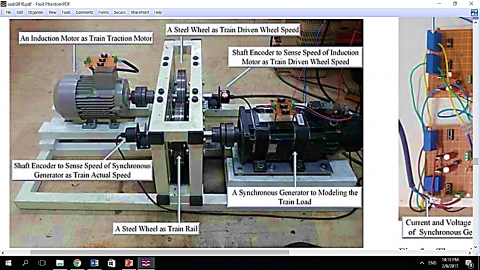Figure 1. Main parts of the test set up 

3.1 General structure of setup

In order to simulate the rail and wheel conditions, two steel wheels are tangent to each other at their contact place. The one, playing the role of the train wheel is coupled from two sides with a shaft encoder and a three-phase induction motor, respectively. This part simulated a traction motor contacted to the train wheel and the driven wheel speed is measured by shaft encoder. The other wheel plays the role of rail and is coupled with a shaft encoder in one side and on the other side, it is coupled with a 3-phase synchronous generator. The speed, sensed by the shaft encoder is equivalent -with the longitudinal velocity of the train. The synchronous generator is connected to a parallel series of electrical resistance, built from light bulbs, representing trainload.

3.2 Speed control strategy

In order to implement the speed control method and adhesion control strategy, a microprocessor is needed. A TMS320F2812 Digital Signal Processor (DSP) is used in the microprocessor part. The main objective of this implemented scheme is to control the adhesion coefficient. In addition to the adhesion control strategy, the inner control loop of the system is speed control of induction motors.

3.3 Field oriented control (FOC)

As it has been mentioned before, in the structure of this setup, the induction motor is used as the traction motor and its speed should be controlled. Induction motor control methods are divided into scalar and vector control. In the presented setup, field-oriented control (FOC), is used to control the induction motor. The scheme of the FOC is shown in Figure2. As it is shown in Figure 2, FOC consists of a torque PI regulator, coordinate transformations, Current regulators and PWM signals generator [26-27]. As the purpose of this study is to identify a linear model for the induction motor in different operating points, the control loops should be opened; therefore d-q voltages (Vds, Vqs) in FOC structure cannot be produced by PI regulators. By designing d-q voltages and applying these two voltages, the motor should be excited. In fact, in this scheme by considering the zero speed condition, the identification of the internal current loop model is carried out by excluding the external loops of speed and flux.

## 2.png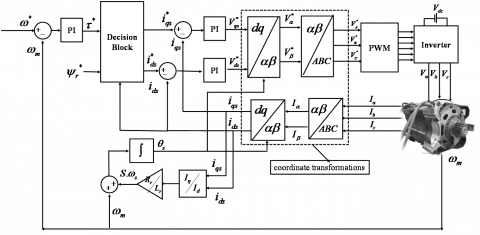Figure 2. Block diagram of the FOC for an induction motor

Firstly, the identification of the induction motor has been done in MATLAB system identification toolbox; using the real parameters of the induction motor, identified in the Advanced Motion Control Laboratory through a VLT Automation Drive FC 300 Danfoss as shown in Figure 3. Based on automatic motor adaptation, the stator resistance Rs, the rotor resistance Rr, the stator leakage inductance L1, the rotor leakage inductance L2, and the main reactance Xh are identified. This parameter cannot be adjusted while the motor is running. After a normal sequence, these parameters are shown on the drive display, shown in Table 1.

## 3.jpgFigure 3. Test setup and VLT automation drive FC300 Danfoss

Table 1. Table title

 Stator Resistance 5.3743 Ω Rotor Resistance 3.5395 Ω Stator Leakage Inductance 3.5530 H Rotor Leakage Inductance 3.5530 H Main Reactance 200.591 Ω

The identified models are two open loop transfer functions which can be substituted in the closed speed control loop. The purpose of opening the loop is to remove the speed control loop in the FOC structure and, apply d-q voltages directly to the induction motor as the inputs in the MATLAB Simulink; therefore the none-controlled angular speed of the induction motor is the output of this simulation. So in this process, in addition to opening the speed control loop, the current regulators are also omitted from the vector control structure. Using the system identification toolbox and having inputs and output vectors of the simulated induction motor (based on real identified parameters), open loop model is extracted. Then, this model is verified based on the real experimental data, measured in the laboratory in the closed-loop FOC system. Regarding the closing loop of the FOC system in the laboratory, the theoretical identified model is validated in the closed-loop FOC system by using real measurements. Using data accusation, the three-phase currents and the speed of the induction motor in the closed-loop system are measured in the laboratory. Therefore, in this approach, by substituting the open loop mathematical model of the induction motor in the closed-loop control system, this theoretical open loop model is validated by using real data of the closed-loop FOC system.

4. Input Design

An important step in the identification process is the design of an appropriate input to excite the system within the frequency range according to the dynamics of the motor. This signal should be Persistently Exciting of an appropriate order. PRBS and PRMS are two important signals in identifying linear and nonlinear models of different systems. The PRBS signal is commonly used to identify linear models and is not sufficiently Persistently Exciting for the nonlinear systems. While the PRMS signal fully identifies a nonlinear system and has many favorable PRBS features [28-30].

PRMS is the multiple-level generalization of GBN (Ground Bayesian Network) of Tulleken (1990) . The amplitude of the PRMS has a uniform distribution over the signal range.

The signal amplitude can have both discrete and continues values in the signal range. $T_{S W}$ is the average switch time of the signal and can be set $T_{s w}=T_{s} / 3$ where Ts is the 98 % settling time . Let be a white noise process with variance $\sigma_{v}^{2}$. A PRMS is obtained by keeping the same (amplitude) value for Ns steps (increasing the block period), i.e,

$u(k)=v\left[\operatorname{int}\left(\frac{k-1}{N_{s}}\right)+1\right], k=1,2, \ldots$    (1)

where int(x) is the integer part of x.

A generalization of this signal is obtained by introducing an additional (random) variable for deciding when to change the level

$u(k)=\left\{\begin{array}{ll}{u(k-1)} & {\text { with probability } \alpha} \\ {u(k)} & {\text { with probability } 1-\alpha}\end{array}\right.$    (2)

It is clear that if a is chosen close to unity, the input will remain constant over long intervals and hence be of low-frequency character. Practical experiences have shown that the best model performance will be achieved if the duration of a change (or hold time) in amplitude is selected as a trade-off between two facts. On the one hand, the duration has to be long enough to cause an effect on the output. On the other hand, too long a time will not give any more dynamic information about the system. Thus, a rule of thumb is: a suitable hold time has to lie close to the settling time of the step response of the system . To deal with this important issue, Pseudo-Random Multilevel Sequences (PRMS) is designed.

The excitation input signal, PRMS is applied to FOC scheme as the DC reference voltages, $v_{d}^{*}, v_{q}^{*}$ which are the current PI regulator outputs as shown in Figure 2 and Figure 4. The angular speed of the induction motor is the output of this open loop system. Using the real measured three phase currents and the angular speed of the induction motor a linear model is identified. The model is evaluated and verified in a closed-loop system, shown in Figure 4.

## 4.png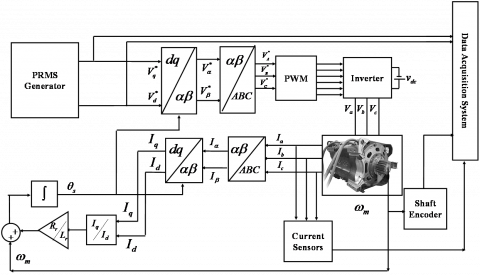Figure 4. Applying of PRMS signal to the IM as the exciting inputs in an open loop system, using real measurements in MATLAB Simulink

4.1 Evaluation of the behavior of three-phase induction motor by applying different inputs

Properly applied inputs play an important role in stimulating the system frequencies. By designing several inputs, we obtained an appropriate range of acceptable frequencies to stimulate the induction motor.

## 5a.png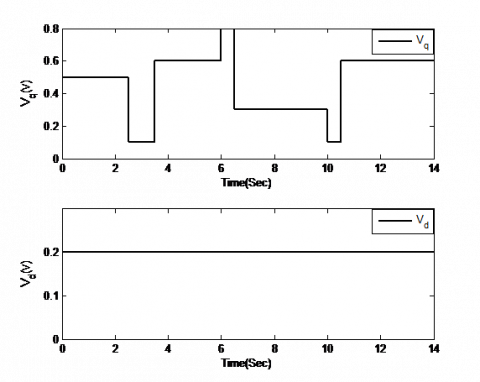（a）

## 5b.png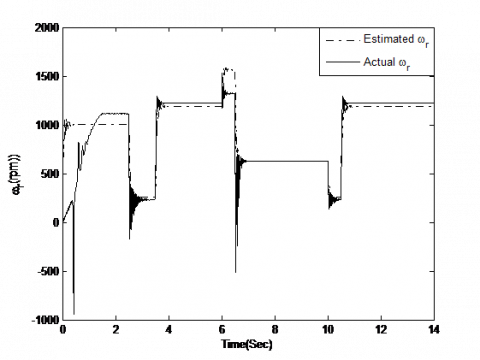（b）

Figure 5. Exciting Inputs, (b) Comparison of the identified model output and real model’s output

## 6a.png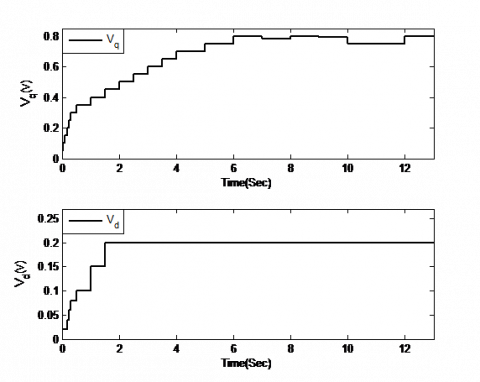（a）

## 6b.png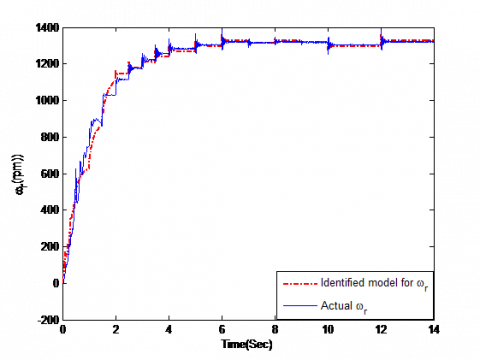（b）

Figure 6. Exciting Inputs, (b) Comparison of the identified model output and real model’s output

## 8888.png## 7b-.pngFigure 7. (a) Exciting Inputs, (b) Comparison of the identified model’s output and real model’s output

As shown in Figure 5, Figure 6 and Figure 7, the induction motor has not been stimulated when input frequencies are greater than 1.5 to 2 Hz, as well as at the moments, when the voltage Vd is variable. Based on the above reasons, these inputs cannot conduce to a correct identified model, so we try to design the input in a way which mostly includes frequencies below 2 Hz and considers Vs constant.

According to what was discussed in the previous sections, Figure 8(a) is an appropriate input for the identification process. In this input design, the Vq has been initially produced at small amplitudes but with high frequencies. After 0.15 seconds, the input range has been fixed and its frequency has increased from 0.75 Hz to 1 Hz. The reason for adopting this method in this input design is to produce a soft signal at the moment of motor start and to consider the acceptable frequency range for better identification of the dynamic model of the motor.

Power Spectral Density (PSD of the exciting input has shown in Figure 9. It tells us where the frequency is lower than 2 Hz, the average power is distributed as a function of frequency.

## 8a.png（a）

## 8b.png（b）

Figure 8. (a) Main exiting input, including frequencies less than 2 Hz, (b) Comparison of the motor and the identified model output

## 9.png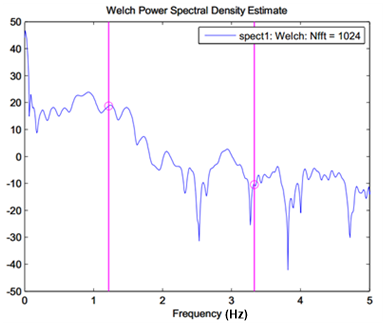Figure 9. Power Spectral Density (PSD) of main designed input

5. Identification Algorithm

After input design, we should choose a model for the three-phase induction motor. Since the induction motor has 5 state variables, we choose the ARMAX model as a mathematical model in the identification process. The Prediction Error Method (PEM) and system identification toolbox of MATLAB software has been used in model identification. Due to the presence of noise in the data acquisition environment and the considered mathematical model, used in the identification process, we used PEM as the identification algorithm. Generally, PEM is one of the system identification methods that calculate the model parameters to provide the best estimation of system output [30, 29].

Using the identification algorithm in the system identification toolbox, we extracted the three-phase induction motor model in an open loop system. As shown in Figure 4, after applying the reference voltages, Vd and Vq as the exciting inputs to the induction motor, the angular speed of the induction motor is measured. Using these inputs and output in the system identification toolbox of MATLAB software, we extracted the mathematical model of the induction motor in an open loop system. The extracted transfer function of the system output (motor angular speed) for Vd and Vq inputs and noise is as follows:

$y=\frac{-1.2 z^{-1}-1.2 z^{-2}}{1-1.463 z^{-1}+1.569 z^{-2}-0.9675 z^{-3}+0.2604 z^{-4}} u_{1}+\frac{0.9006 z^{-1}+0.4277 z^{-2}}{1-1.463 z^{-1}+1.569 z^{-2}-0.9675 z^{-3}+0.2604 z^{-4}} u_{2}+\frac{1-0.301 z^{-1}+0.2484 z^{-2}}{1-1.463 z^{-1}+1.569 z^{-2}-0.9675 z^{-3}+0.2604 z^{-4}} e$                                            (3)

In order to simplify the calculation, the model can be reduced to a lower order. Thus, with the elimination of the non-dominant pole, we have:

$\omega_{r}=\frac{0.07609 z^{3}-1.529 z^{2}-1.419 z-1.702}{z^{3}-0.8261 z^{2}+0.9281 z-0.3417} u_{1}+\frac{-0.03647 z^{3}+1.062 z^{2}+0.7444 z+0.8549}{z^{3}-0.8118 z^{2}+0.936 z-0.3358} u_{2}+\frac{1.313 z^{2}+0.8147 z+1.51}{z^{2}-0.06238 z+0.594} e$                                            (4)

Regarding Figure 4, The d-q currents can be shown as follows:

## 10.png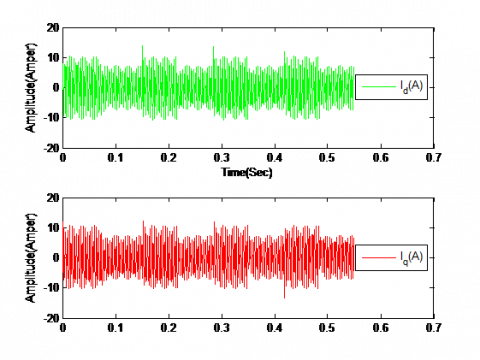Figure 10. d-q currents generated in the IM simulation in MATLAB Simulink

By data sampling of the above currents, Figure 10 and considering them in the MATLAB system identification tool, the voltage-current transfer function derives based on the ARMAX model. The output conformance of these models and the IM simulation, which generates the d-q currents are shown in Figure 11.

## 11a.png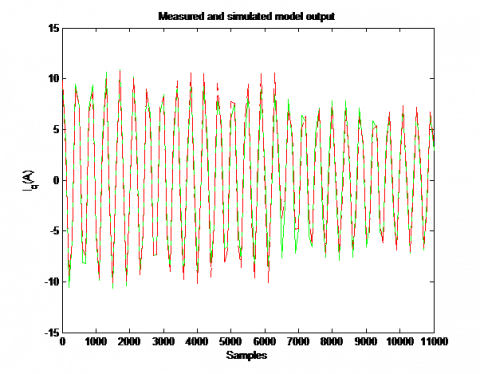（a）

## 11b.png（b）

Figure 11. Exciting Inputs, (b) Comparison of the identified model output and real model’s output

The identified models for the current-voltage transfer functions after order reduction are as follows:

(1). Identified transfer function for Voltage-d Current:

$I_{d}=\frac{0.002301 z^{2}-0.0003886 z+0.003027}{z^{2}-0.142 z+0.9968} u_{1}+\frac{0.005495 z^{2}+0.007699 z-0.01436}{z^{2}-0.142 z+0.9968} u_{2}+\frac{1.641 z^{2}-0.6641 z+1.248}{z^{2}-0.142 z+0.9968} e$                                                 (5)

(2). Identified transfer function for Voltage-Q current:

$I_{q}=\frac{-0.0001422 z^{2}-0.001546 z-0.001974}{z^{2}-0.1407 z+0.9968} u_{1}+\frac{-0.001091 z^{2}+0.01722 z-0.01515}{z^{2}-0.1407 z+0.9968} u_{2}+\frac{1.046 z^{2}+0.01544 z+0.6445}{z^{2}-0.1407 z+0.9968} e$                                               (6)

By replacing the identified models in the structure of Figure 2, the block diagrams of the induction motor with its speed control loop changes to the simplified closed-loop speed control system Figure 12.

## 12.pngFigure 12. Power Spectral Density (PSD) of main designed input

6. Validation

Validation of this model is performed in order to determine whether the extracted model is credible or not. A common way of validation is to compare the output of the extracted model with the real system output by the same inputs. In system identification, model validation involves testing of the residuals which is the difference between measurements and the system model outputs. When the extracted model properly describes the real system behavior, the residuals contain only observation noise. In this section, the open loop identified model has been replaced in the closed-loop structure and using the real data measurements, obtained from the closed-loop system in the Advanced Motion Control Laboratory, the open-loop extracted model is verified.

6.1 Autocorrelation and cross-correlation band consideration

In Figure 13 the autocorrelation of the residual of the first and second channel outputs and the cross-correlation between the input and output of the first and second channels for the speed transfer function is in the three-sigma limits is shown. Since the autocorrelation is not extracted from this band, the identification is done correctly.

## 13a.png（a）

## 13b.png（b）

Figure 13. Autocorrelation of the residual of the first and second channel outputs and the cross- correlation between the input and output of the first and second channels

6.2 Validation based on real measurements

In order to validate the extracted model based on the real parameters of the induction motor, data acquisition and measurements of the real induction motor has been dealt with the advanced motion control laboratory. The motor currents and speed has been measured by using the NI-CRIO data logger.

## 14-3.pngFigure 14. Real data acquisition in the laboratory

## 15.jpgFigure 15. Real data acquisition in the laboratory

6.2.1 Consideration of the identified model using real measurements

In this section real data of the induction motor seed is converted from a pulse to a continuous values, then by applying the actual values of the three-phase currents and the d-q voltages to the identified model, the motor speed could be obtained from the identified model, and finally, with the real speed of the comparison engine.

6.2.2 Comparison of the actual speed of the motor with the speed of the identified model

By applying the mentioned method, the shaft encoder pulses convert to the angular speed of the induction motor which is 1000 rpm in the steady state. By employing the real measured currents in the laboratory and random d-q voltages to the identified model, the angular speed of the motor reaches to 1000 rpm in the steady state, showing in Figure 16.

## 16.pngFigure 16. Comparison of the real speed of the induction motor with the speed of the identified model

7. Conclusions

In this paper, theoretical simulations and practical datasets were developed to extract a linear model of a three-phase induction motor. In this process, firstly, by examining the different inputs for different frequencies, the engine performance was examined, and then the engine speed was obtained by selecting the best input which had sufficient enough PE and also the optimal frequency range. By determining the appropriate input, the time and frequency characteristics of the model were obtained and also statistical characteristics such as Auto-correlation and cross-correlation were investigated. In the next step, the identification process and the identified model were verified by performing real experimental data mapping to the obtained model. In other words, the identification process was performed by closing the loop of speed control in the vector control structure. Subsequently, the model derived from the theoretical simulations in an open loop identification process was validated and verified by measured real experimental data from the real closed-loop system.

References

 Borrelli, F., Bemporad, A., Fodor, M., Hrovat, D. (2006). An MPC/hybrid system approach to traction control. IEEE Transactions on Control Systems Technology, 14(3): 541–552. https://doi.org/10.1109/TCST.2005.860527

 Sun, H., Hou, Z., Tang, T. (2011). An iterative learning approach for train trajectory tracking control 1. IFAC Proceedings Volumes, 44(1): 14916–14921. https://doi.org/10.3182/20110828-6-it-1002.01270

 Cai, W.C., Li, D.Y., Song, Y.D. (2015). A novel approach for active adhesion control of high-speed trains under antiskid constraints. IEEE Transactions on Intelligent Transportation Systems, 16(6): 3213–3222. https://doi.org/10.1109/TITS.2015.2440654

 Henke, M., Narjes, G., Hoffmann, J., Wohlers, C., Urbanek, S., Heister, C., Steinbrink, J., Canders, W.R., Ponick, B. (2018). Challenges and opportunities of very light high-performance electric drives for aviation. Energies, 11(2): 344. https://doi.org/10.3390/en11020344

 Yaacob, S., Mohamed, F.A. (1998). Black-box modeling of the induction motor. in SICE’98. Proceedings of the 37th SICE Annual Conference. International Session Papers, 883–886. https://doi.org/10.1109/SICE.1998.742935

 Toliyat, H.A. Levi, E., Raina, M. (2003). A review of RFO induction motor parameter estimation techniques. IEEE Transactions on Energy Conversion, 18(2): 271-283. https://doi.org/10.1109/TEC.2003.811719

 Jeong, S.G., Lee, B.S., Kim, K.S., Park, M.H. (1991). The analysis and compensation of dead-time effects in PWM inverters. Industrial Electronics Society, 38(2): 108–114. https://doi.org/10.1109/IECON.1988.665770

 Sukegawa, T., Kamiyama, K., Matsui, T., Okuyama, T. (1988). Fully digital, vector-controlled PWM VSI-fed ac drives with an inverter dead-time compensation strategy. IEEE Transactions on Industry Applications, 463–469. https://doi.org/10.1109/28.81841

 Lee, S.H., Yoo, A., Lee, H.J., Yoon, Y.D., Han, B.M. (2017). Identification of induction motor parameters at standstill based on integral calculation. IEEE Transactions on Industry Applications, 53(3): 2130–2139. https://doi.org/10.1109/TIA.2017.2650141

 Kwon, W.H., Lee, C.H., Youn, K.S., Cho, G.H. (1994). Measurement of rotor time constant taking into account magnetizing flux in the induction motor. Industry Applications Society Annual Meeting, 1: 88–92. https://doi.org/10.1109/IAS.1994.345494

 Aminu, M., Ainah, P.K., Abana, M., Abu, U.A. (2018). Identification of induction machine parameters using only no-load test measurements. Nigerian Journal of Technology, 37(3): 742–748.

 Cirrincione, M., Pucci, M. (2002). Experimental verification of a technique for the real-time identification of induction motors based on the recursive least-squares. Advanced Motion Control, 2002. 7th International Workshop, pp. 326–334. https://doi.org/10.1109/AMC.2002.1026940

 Stephan, J., Bodson, M., Chiasson, J. (1994). Real-time estimation of the parameters and fluxes of induction motors. IEEE Transactions on Industry Applications, 30(3): 746–759. https://doi.org/10.1109/28.293725

 Mapelli, F.L., Bezzolato, A., Tarsitano, D. (2012). A rotor resistance MRAS estimator for induction motor traction drive for electrical vehicles. Electrical Machines (ICEM), 2012 XXth International Conference, pp. 823-829. https://doi.org/10.1109/ICElMach.2012.6349972

 Maiti, S., Chakraborty, C., Hori, Y., Ta, M.C. (2008). Model reference adaptive controller-based rotor resistance and speed estimation techniques for vector controlled induction motor drive utilizing reactive power. IEEE Transactions on Industrial Electronics, 55(2): 594–601. https://doi.org/10.1109/TIE.2007.911952

 Baneira, F., Yepes, A.G., López, O., Doval-Gandoy, J. (2016). Estimation method of stator winding temperature for dual three-phase machines based on dc-signal injection. IEEE Transactions on Power Electronics, 31(7): 5141–5148. https://doi.org/10.1109/TPEL.2015.2479410

 Abdelhadi, B., Benoudjit, A., Nait-Said, N. (2005). Application of genetic algorithm with a novel adaptive scheme for the identification of induction machine parameters. IEEE Transactions on Energy Conversion, 20(2): 284–291. https://doi.org/10.1109/TEC.2004.841508

 Castaldi, P., Geri, W., Montanari, M., Tilli, A. (2005). A new adaptive approach for on-line parameter and state estimation of induction motors. Control Engineering Practice, 13(1): 81–94. https://doi.org/10.1016/j.conengprac.2004.02.008

 Delfine, L., Salvatore, L. (2005). Grey-box identification of induction motor model for field oriented control. Industrial Electronics, 2005. ISIE 2005. Proceedings of the IEEE International Symposium, 1: 13–18. https://doi.org/10.1109/ISIE.2005.1528881

 Arrofiq, M., Saad, N., Karsiti, M.N. (2009). An Identification of model for integrated variable speed drive, induction motor and dynamometer. Industrial Electronics & Applications, 2009. ISIEA 2009. IEEE Symposium on, 1: 337–342. https://doi.org/10.1109/ISIEA.2009.5356457

 Mohamed, F.A., Koivo, H. (2004). Modeling of induction motor using non-linear neural network system identification. SICE 2004 Annual Conference, 2: 977–982.

 Saleem, A., Issa, R., Tutunji, T. (2010). Hardware-in-the-loop for on-line identification and control of three-phase squirrel cage induction motors. Simulation Modelling Practice and Theory, 18(3): 277–290. https://doi.org/10.1016/j.simpat.2009.11.002

 Akbari, A., Rahbar, K., Taghiabad, M.J.M. (2006). Induction motor identification using Elman neural network. WSEAS Trans. Syst, 5(4): 766–770.

 Elman, J.L. (1990). Finding structure in time. Cognitive Science, 14(2): 179–211. https://doi.org/10.1207/s15516709cog1402_1

 Sadr, S., Khaburi, D.A., Rivera, M. (2016). A new hardware device to simulate the movement of electric train wheel on rail. 2016 7th Power Electronics and Drive Systems Technologies Conference (PEDSTC), pp. 87–92. https://doi.org/10.1109/PEDSTC.2016.7556843

 Casadei, D., Profumo, F., Serra, G., Tani, A. (2002). FOC and DTC: Two viable schemes for induction motors torque control. Converter Technology & Electric Traction, 17(5): 779–787. https://doi.org/10.1109/TPEL.2002.802183

 Ohtani, T., Takada, N., Tanaka, K. (1992). Vector control of induction motor without shaft encoder. IEEE Transactions on Industry Applications, 28(1): 157–164. https://doi.org/10.1109/IAS.1989.96697

 Nowak, R.D., Van Veen, B.D. (1993). Nonlinear system identification with pseudorandom multilevel excitation sequences. Acoustics, Speech, and Signal Processing, 1993. ICASSP-93., 1993 IEEE International Conference on, 4: 456–459. https://doi.org/10.1109/ICASSP.1993.319693

 Zhu, Y. (2001). Multivariable system identification for process control. Elsevier. https://doi.org/10.1016/b978-008043985-3/50011-9

 van den Bosch, P.P.J., van der Klauw, A.C. (1994). Modeling, identification and simulation of dynamical systems. CRC Press.

 Tulleken, H.J.A.F. (1990). Generalized binary noise test-signal concept for improved identification-experiment design. Automatica, 26(1): 37–49. https://doi.org/10.1016/0005-1098(90)90156-c

 Zhu, Y.C. (2000). Identification of Hammerstein models for control using ASYM. International Journal of Control, 73(18): 1692–1702. https://doi.org/10.1080/00207170050201771

 Jelali, M., Kroll, A. (2012). Hydraulic servo-systems: modeling, identification and control. Springer Science & Business Media. https://doi.org/10.1007/978-1-4471-0099-7

 Arabaci, H., Bilgin, O. (2012). A novel motor speed calculation method using square wave speed sensor signals via fast Fourier transform. Turkish Journal of Electrical Engineering and Computer Sciences, 20(1): 1090–1099.